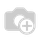# Understanding OpenERP domain filter?Explaination of OpenERP domain filter with simple example:

Consider the following fields in XML view.

<field name="field1"/>

<field name="field2"/>

<field name="field3"/>

Single condition:

Simple condition in programming:

if field1 = 10

In OpenERP domain filter, it will be written as:

syntax : Each tuple in the domain has three fields ->  ('field_name', 'operator', value)

field_name : a valid name of field of the object model or in the database table

operator : valid operators are =, !=, >, >=, <, <=, like, ilike, in, not in, child_of, parent_left, parent_right (openerp/osv/expression.py)

value : a valid value to compare with the values of field_name, depending on its type.

ie, domain = [('field1','=',10)] # where field1 should be a field in the model and 10 will be the value

or domain = [('field1','=',field2)] # where field1 and field2 should be the fields in the model

Condition AND

Simple condition in programming:

if field1 = 5 and field2 = 10

In OpenERP domain filter, it will be written as:

ie, domain = [('field1','=',5),('field2','=',10)]

or domain = [('field1','=',field3),('field1','=',field3)]

Note : Note that if you don't specify any condition at the beginning and condition will be applied.

Condition OR

Simple condition in programming:

if field1 = 5 or field2 = 10

In OpenERP domain filter, it will be written as:

ie, domain = ['|', ('field1','=',5),('field2','=',10)]

or domain = ['|', ('field1','=',field3),('field1','=',field3)]

Multiple Condition

Simple condition in programming:

if field1 = 5 or (field2 ! = 10 and field3 = 12)

In OpenERP domain filter, it will be written as:

domain = ['|',('field1','=',5),('&',('field2','!=',10),('field3','=','12'))]

Also this post from Vivek will be helpful to all. Cheers!!Hi there, thank you for the explanation. Can you help me to understand this domain filter for contracts: [('employee_id.user_id', 'in', [usr.id for usr in user.user_ids])] Thank you in advance.

I can't understand that s*** sorry! Help needed!

['&', '|',

('product_id','=',product_id),

'&',

('product_tmpl_id.product_variant_ids','=',product_id),

('product_id','=',False),

('type', '=', 'normal')]

Hi Muhammad, that code can be interpreted as:

(('product_id','=',product_id) or (('product_tmpl_id.product_variant_ids','=',product_id) and ('product_id','=',False))) and ('type', '=', 'normal')

keep in mind the order of parentheses.

HI, thenk you for the explanation.

Can you help me, i want to do the filtering with a simple condition but using a value of the function type field

see the code

gerant_structure_id = fields.Integer('Structure id', compute='_getStructureIdForCurrentUser')

@api.one
def _getStructureIdForCurrentUser(self):
structure_id = self.env['afya.structure.sante'].search([('gerant_id', '=', self.env.uid )]).id
self.gerant_structure_id = structure_id

view ...

<record model="ir.actions.act_window" id="compte_wallet_list_action">
<field name="name">Compte_Wallet</field>
<field name="domain">[('structure_sante_id', '=',  gerant_structure_id)]</field>
<field name="res_model">afya.compte.wallet</field>
<field name="view_mode">tree</field>

</record>I'm looking for some insight about a tiny detail:

Regarding this definition

domain = ['|',('field1','=',5),('&',('field2','!=',10),('field3','=','12'))]

what's/why the difference between the lack of quotes like in 5 or 10 values and and the quoted one like in '12' ?

Thanks

1 CommentThe coder may declared the fields3 as string fields and other 2 fields as integer